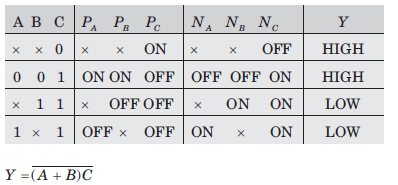Courses

# Test: Microprocessor & Digital Logic Families

## 15 Questions MCQ Test GATE ECE (Electronics) 2022 Mock Test Series | Test: Microprocessor & Digital Logic Families

Description
This mock test of Test: Microprocessor & Digital Logic Families for Railways helps you for every Railways entrance exam. This contains 15 Multiple Choice Questions for Railways Test: Microprocessor & Digital Logic Families (mcq) to study with solutions a complete question bank. The solved questions answers in this Test: Microprocessor & Digital Logic Families quiz give you a good mix of easy questions and tough questions. Railways students definitely take this Test: Microprocessor & Digital Logic Families exercise for a better result in the exam. You can find other Test: Microprocessor & Digital Logic Families extra questions, long questions & short questions for Railways on EduRev as well by searching above.
QUESTION: 1

### Consider the following program of 8085 assembly language: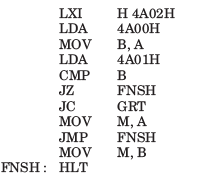If the contents of memory location 4A00H, 4A01H and 4A02H, are respectively A7H, 98H and 47H, then after the execution of program contents of memory location 4A02H will be respectively

Solution: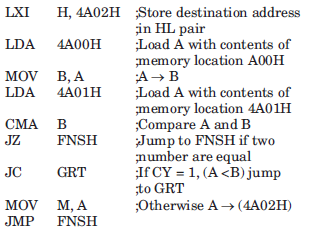GRT : MOV M, B
FNSH : HLT

This program find the larger of the two number stored in location 4A00H and 4A01H and store it in memory location 4A002.

A7H > 98H Thus A7H will be stored at 4A02H.

QUESTION: 2

### Consider the following program of 8085 assembly language:Que: The memory requirement for this program is

Solution:

Operand R, M or implied : 1–Byte instruction
Operand 8–bit : 2–Byte instruction
Operand 16–bit : 3–Byte instruction

3–Byte instruction are: LXI, LDA, JZ, JC, JMP
P–Byte instruction are : MOV, CMP, HLT

Hence memory = 3 x 6 + 1 x 5 = 23 bytes

QUESTION: 3

### The instruction, that does not clear the accumulator of 8085, is

Solution:

All instruction clear the accumulator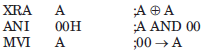QUESTION: 4

Consider the following loop

XRA     A
LXI       B, 0007H
LOOP : DCX     B
JNZ      LOOP

This loop will be executed

Solution:

The instruction XRA will set the Z flag. LXI and DCX does not alter the flag. Hence this loop will be executed 1 times.

QUESTION: 5

How many address bits are needed to select all memory locations in the 2118 16K × 1 RAM?

Solution:
QUESTION: 6

The contents of accumulator after the execution of following instruction will be

MVI      A, A7H
ORA    A
RLC

Solution: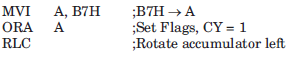The contents of bit D7 are placed in bit D0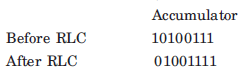QUESTION: 7

The contents of accumulator after the execution of following instructions will be

MVI      A, B7H
ORA     A
RAL

Solution:

RAL instruction rotate the accumulator left through carry.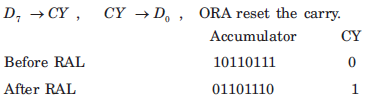QUESTION: 8

The contents of the accumulator after the execution of the following program will be

MVI        A, C5H
ORA       A
RAL

Solution:

RRC instruction rotate the accumulator right and D0 is placed in D7 .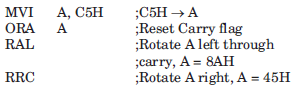QUESTION: 9

Consider the following set of instruction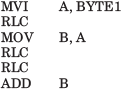If BYTE1 = 07H, then content of A, after the execution of program will be

Solution:

This program multiply BYTE1 by 10. Hence content of A will be 46H.

07H = 0710 , 7 x 10 = 70, 7010 = 46H

QUESTION: 10

Consider the following program

MVI      A,BYTE1
RRC
RRC

If BYTE1 = 32H, the contents of A after the execution of program will be

Solution:

Contents of Accumulator A = 0011 0010

After First RRC = 0001 1001
After second RRC = 1000 1100

QUESTION: 11

The circuit shown in fig. is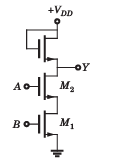Solution:

If either one or both the inputs are V(0) = 0 V the corresponding FET will be OFF, the voltage across the load FET will be 0 V, hence the output is VDD . If boths inputs are V(1) = VDD, both M1 and M2 are ON and the output is V(0) = 0 V. It satisfy NAND gate.

QUESTION: 12

The circuit shown in fig. acts as a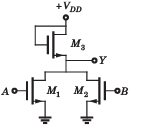Solution:

If both the inputs are at V(0) = 0 V, the transistor M1 and M2 are OFF, hence the output is V(1) = VDD  . If either one or both of the inputs are at V(1) = VDD  , the corresponding FET will be ON and the output will be V (0) = 0 V. Hence it is a NOR gate.

QUESTION: 13

The circuit shown in fig.  implements the function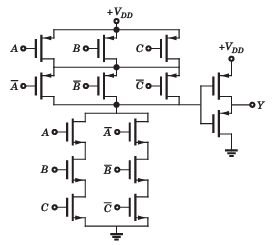Solution:

If all inputs A, B and C are HIGH, then input to invertor is LOW and output Y is HIGH. If all inputs are LOW, then input to inverter is also LOW and output Y is HIGH. In all other case the input to inverter is HIGH and output Y is LOW.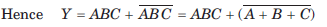QUESTION: 14

The circuit shown in fig. implements the function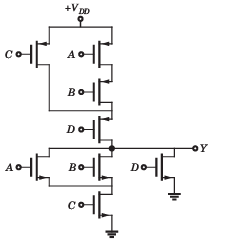Solution:

The operation of circuit is given below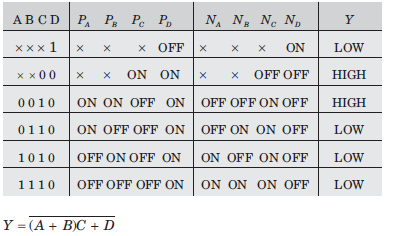QUESTION: 15

Consider the CMOS circuit shown in fig. The output Y is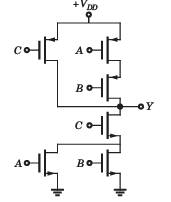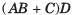Solution:

The operation of this circuit is given below :